# Calculus Without Tears

### Computation

#### The FDM substitutions

The solution for a time dependent 2-D problem is computed for a set of grid points that cover the problem domain, a representative grid is shown in the diagram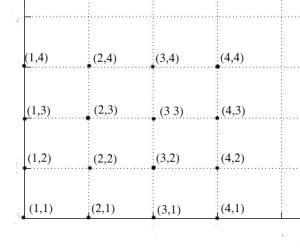The model for time dependent 2-D heat transfer is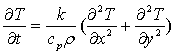Note that temperature in the plate is a function of three variables, T(t, x, y), and taking samples at discrete times and grid points gives values for T(n, i, j). The standard notation for the sampled values is Tn(i,j).

We make the usual Euler's substitution for the time derivative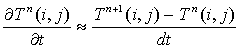The FDM substitutions for the spatial derivatives are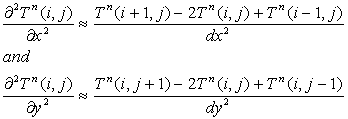Making both substitutions into the differential equation model gives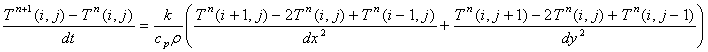and we can rearrage the equation to solve for Tn+1(i,j)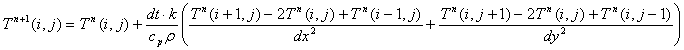and we're good to go.

#### The code

With dx = dy and C = dt*k / (cp*p*dx2), the code is

for n = 1:N
Tn = T; % save Tn before calculating Tn+1
for i = 2:sizeX - 1 % note that boundary points are omitted
for j = 2:sizeY - 1
T(i,j) = T(i,j) + C*(Tn(i+1,j) + Tn(i-1,j) + Tn(i,j+1) + Tn(i,j-1) - 4*T(i,j));
end
end
% apply boundary conditions here
end

Simple as that !